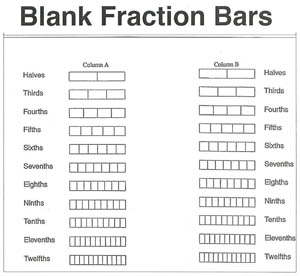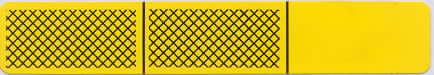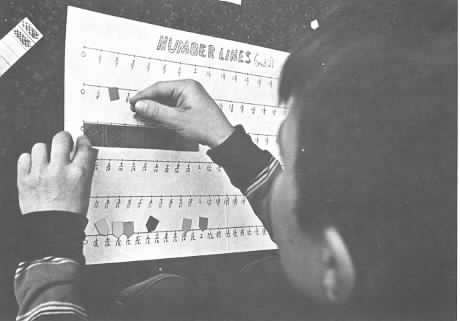Home
 Inequality of FractionsMaterials: Sheets of Blank Fraction Bars Inequality can be modeled by comparing the shaded amounts of bars. This will help to show that the basic idea of inequality is the same for fractions as for whole numbers, that is, comparing two amounts to see which is greater.2/3 < 3/4 Select from the following pairs of fractions and ask students to discuss methods for determining an inequality for each pair. A variety of methods can be used (see below). Discuss some of these.Some of the above inequalities can be determined by comparing them to 1/2. For example, 5/9 is greater than 1/2 because more than half of its bar is shaded and 3/8 is less than 1/2 because less than half of its bar is shaded. A visual model can be used to explain why 1/11 is less than 1/8. When a whole is divided into 11 equal parts the parts are smaller than when it is divided into 8 equal parts. Similarly, 7/8 is closer to a whole than 4/5, because 7/8 is missing only 1/8, whereas 4/5 is missing 1/5 which is greater than 1/8. Some of the above pairs of fractions will require one of the standard rules for determining inequalities, such as obtaining a common denominator or cross multiplying. NCTM Standards 2000 It is important to encourage students to use visual models even after fraction symbols have been introduced. Standards 2000, page 150, notes that if students have a visual model, then comparing fractions to benchmarks like 1/2 and 1 becomes easy. For further activities involving inequality of fractions use Blank Fraction Bars together with the following three activity sheets: Shading Blank Fraction Bars to See Inequalities; and Shading Blank Fraction Bars to See Equality and Inequality; and Shading Blank Fraction Bars to See Inequality Relationships. One of the activities on the Number Lines mat is to place markers for equalities and inequalities of fractions. Side 2 of the mat is shown below and has fractions for fourths, fifths, sixths, eighths, tenths, and twelfths.Photo courtesy of Herb Moyer